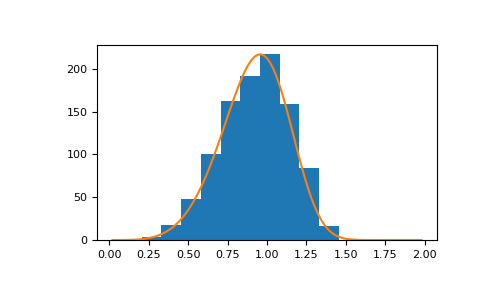# numpy.random.mtrand.RandomState.weibull¶

method

RandomState.weibull(a, size=None)

Draw samples from a Weibull distribution.

Draw samples from a 1-parameter Weibull distribution with the given shape parameter a.Here, U is drawn from the uniform distribution over (0,1].

The more common 2-parameter Weibull, including a scale parameteris just.

Parameters: a : float or array_like of floats Shape parameter of the distribution. Must be nonnegative. size : int or tuple of ints, optional Output shape. If the given shape is, e.g., (m, n, k), then m * n * k samples are drawn. If size is None (default), a single value is returned if a is a scalar. Otherwise, np.array(a).size samples are drawn. out : ndarray or scalar Drawn samples from the parameterized Weibull distribution.

Notes

The Weibull (or Type III asymptotic extreme value distribution for smallest values, SEV Type III, or Rosin-Rammler distribution) is one of a class of Generalized Extreme Value (GEV) distributions used in modeling extreme value problems. This class includes the Gumbel and Frechet distributions.

The probability density for the Weibull distribution iswhereis the shape andthe scale.

The function has its peak (the mode) at.

When a = 1, the Weibull distribution reduces to the exponential distribution.

References

  Waloddi Weibull, Royal Technical University, Stockholm, 1939 “A Statistical Theory Of The Strength Of Materials”, Ingeniorsvetenskapsakademiens Handlingar Nr 151, 1939, Generalstabens Litografiska Anstalts Forlag, Stockholm.
  Waloddi Weibull, “A Statistical Distribution Function of Wide Applicability”, Journal Of Applied Mechanics ASME Paper 1951.
  Wikipedia, “Weibull distribution”, https://en.wikipedia.org/wiki/Weibull_distribution

Examples

Draw samples from the distribution:

>>> a = 5. # shape
>>> s = np.random.weibull(a, 1000)


Display the histogram of the samples, along with the probability density function:

>>> import matplotlib.pyplot as plt
>>> x = np.arange(1,100.)/50.
>>> def weib(x,n,a):
...     return (a / n) * (x / n)**(a - 1) * np.exp(-(x / n)**a)

>>> count, bins, ignored = plt.hist(np.random.weibull(5.,1000))
>>> x = np.arange(1,100.)/50.
>>> scale = count.max()/weib(x, 1., 5.).max()
>>> plt.plot(x, weib(x, 1., 5.)*scale)
>>> plt.show()#### Previous topic

numpy.random.mtrand.RandomState.wald

#### Next topic

numpy.random.mtrand.RandomState.zipf